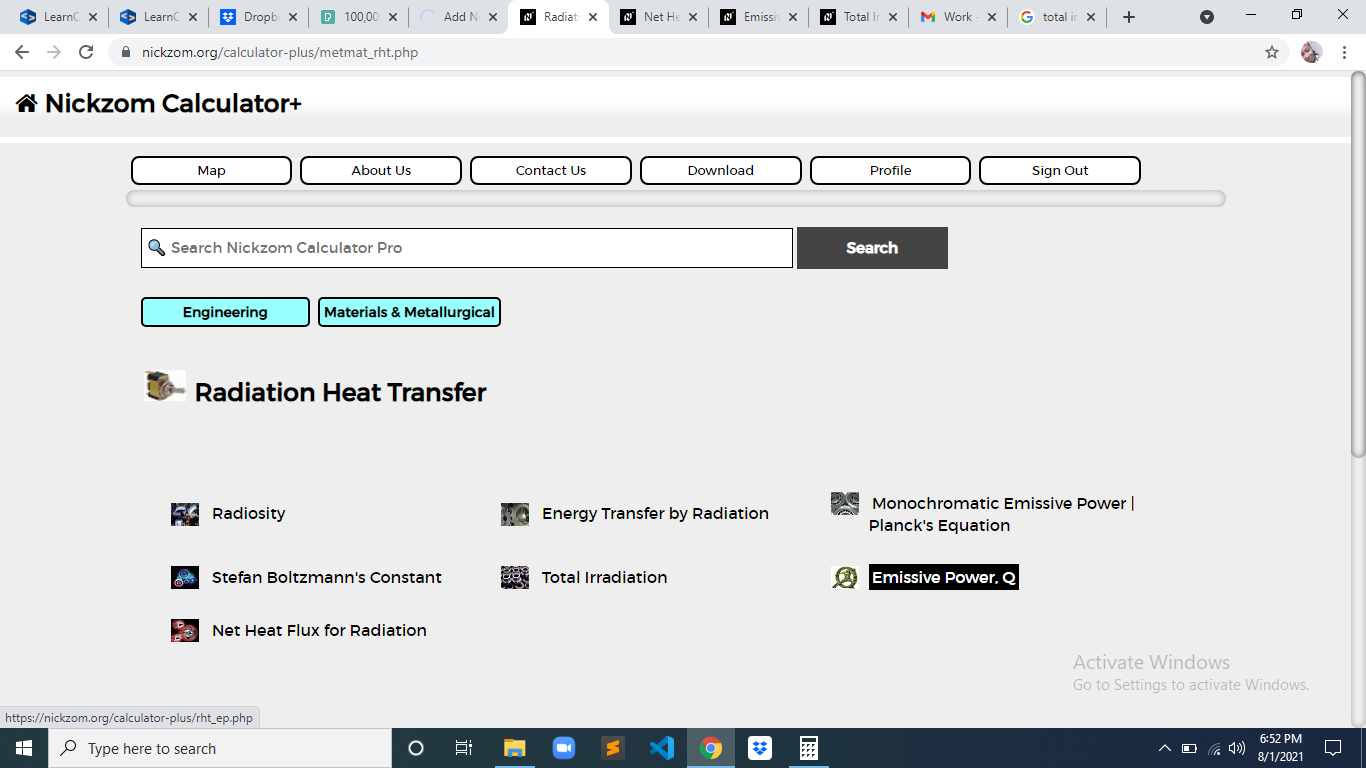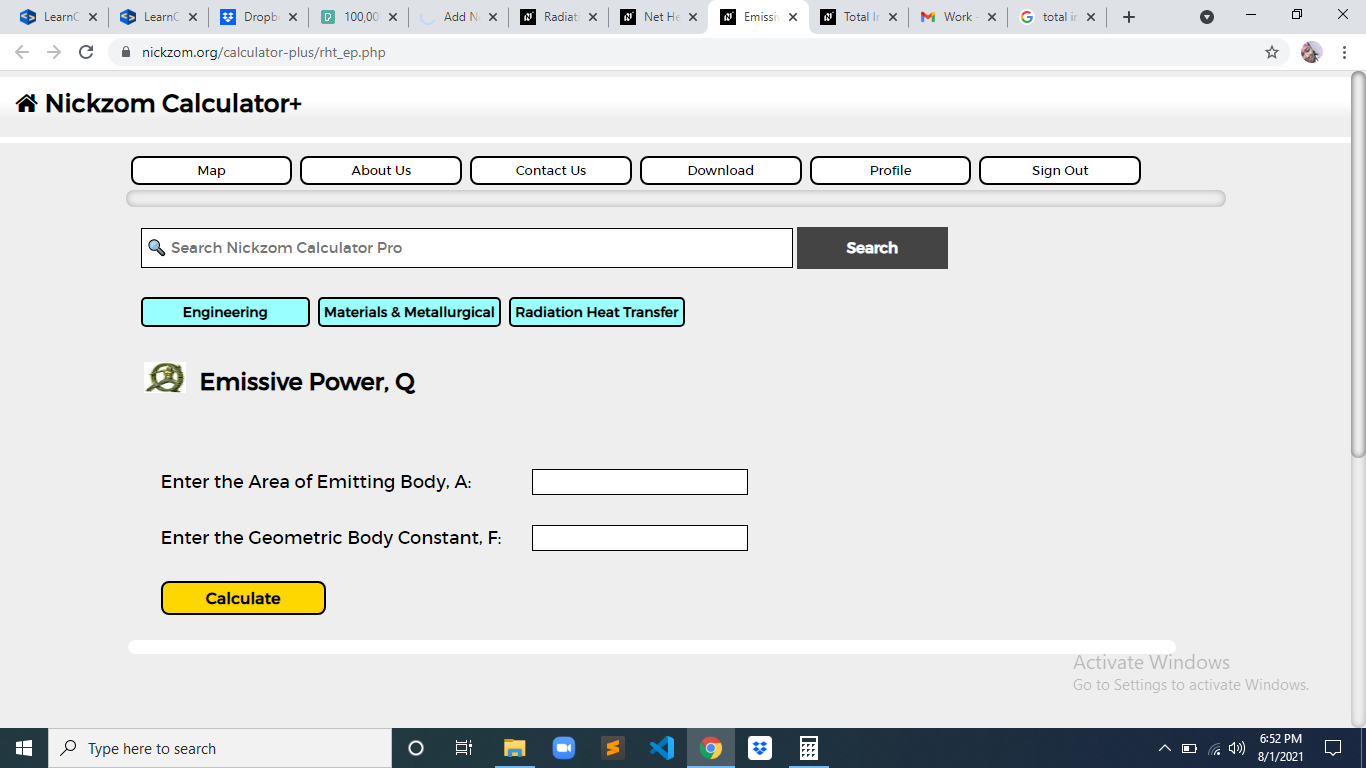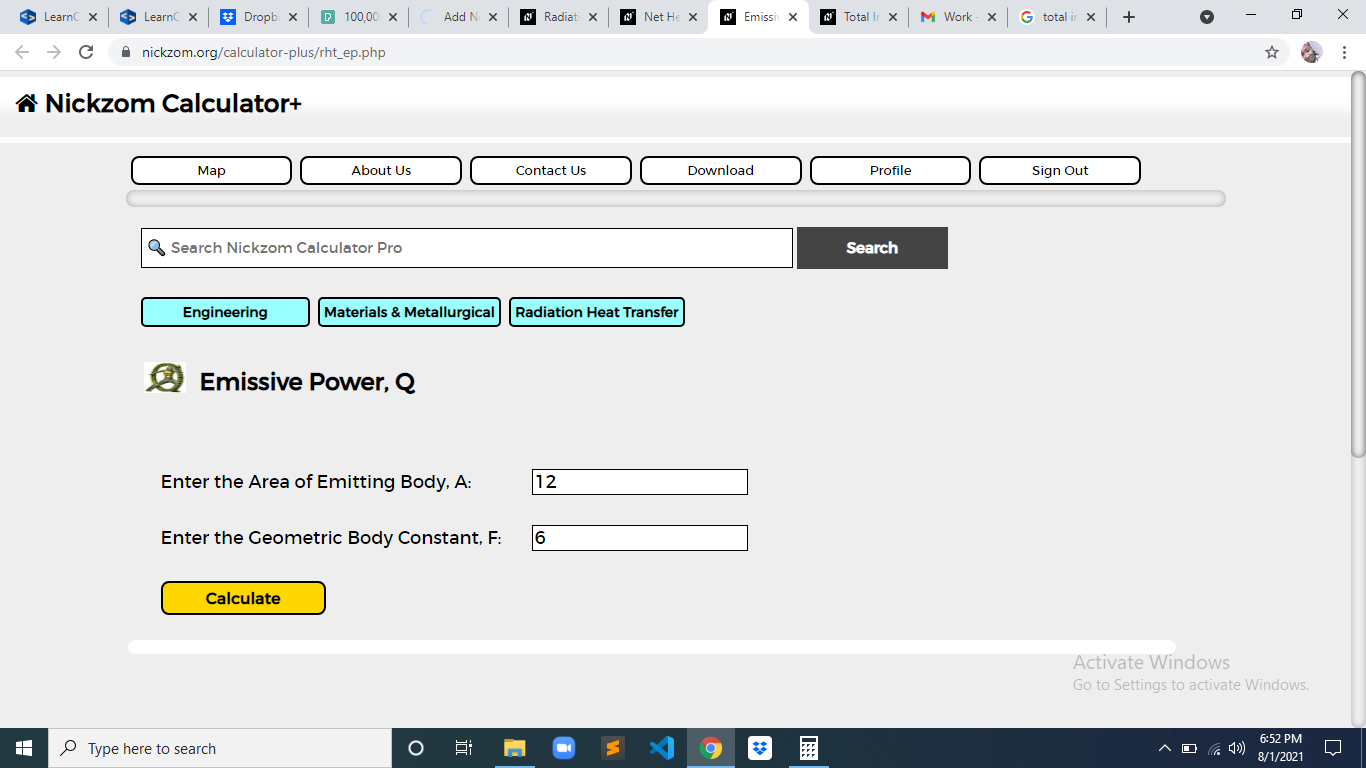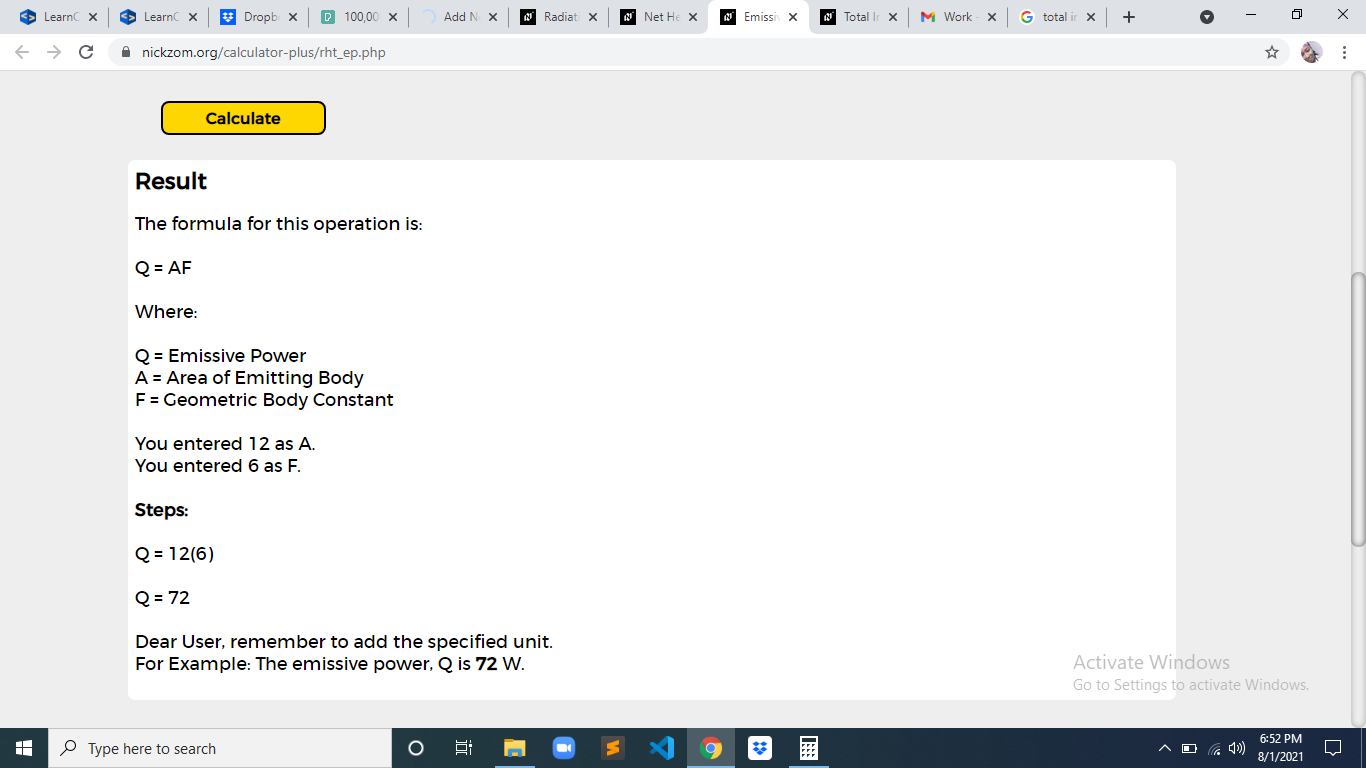# How to Calculate and Solve for Emissive Power | Radiation Heat TransferThe image above represents emissive power.

To compute for emissive power, two essential parameters are needed and these parameters are Area of Emitting Body (A) and Geometric Body Constant (F).

The formula for calculating emissive power:

Q = AF

Where:

Q = Emissive Power
A = Area of Emitting Body
F = Geometric Body Constant

Let’s solve an example;
Find the emissive power when the area of emitting body is 12 and the geometric body constant is 6.

This implies that;

A = Area of Emitting Body = 12
F = Geometric Body Constant = 6

Q = AF
Q = 12(6)
Q = 72

Therefore, the emissive power is 72 W.

Calculating the Area of Emitting Body when the Emissive Power and the Geometric Body Constant is Given.

A = Q / F

Where:

A = Area of Emitting Body
Q = Emissive Power
F = Geometric Body Constant

Let’s solve an example;
Find the area of emitting body when the emissive power is 32 and the geometric body constant is 8.

This implies that;

Q = Emissive Power = 32
F = Geometric Body Constant = 8

A = Q / F
A = 32 / 8
A = 4

Therefore, the area of emitting body is 4.

Calculating the Geometric Body Constant when the Emissive Power and the Area of Emitting Body is Given.

F = Q / A

Where:

F = Geometric Body Constant
Q = Emissive Power
A = Area of Emitting Body

Let’s solve an example;
Find the geometric body constant when the emissive power is 36 and the area of emitting body is 12.

This implies that;

Q = Emissive Power = 36
A = Area of Emitting Body = 12

F = Q / A
F = 36 / 12
F = 3

Therefore, the geometric body constant is 3.

Nickzom Calculator – The Calculator Encyclopedia is capable of calculating the emissive power.

To get the answer and workings of the emissive power using the Nickzom Calculator – The Calculator Encyclopedia. First, you need to obtain the app.

You can get this app via any of these means:

To get access to the professional version via web, you need to register and subscribe for NGN 2,000 per annum to have utter access to all functionalities.
You can also try the demo version via https://www.nickzom.org/calculator

Apple (Paid) – https://itunes.apple.com/us/app/nickzom-calculator/id1331162702?mt=8
Once, you have obtained the calculator encyclopedia app, proceed to the Calculator Map, then click on Materials and Metallurgical under Engineering.Now, Click on Radiation Heat Transfer under Materials and MetallurgicalNow, Click on Emissive Power under Radiation Heat TransferThe screenshot below displays the page or activity to enter your values, to get the answer for the emissive power according to the respective parameter which is the Area of Emitting Body (A) and Geometric Body Constant (F).Now, enter the values appropriately and accordingly for the parameters as required by the Area of Emitting Body (A) is 12 and Geometric Body Constant (F) is 6.Finally, Click on CalculateAs you can see from the screenshot above, Nickzom Calculator– The Calculator Encyclopedia solves for the emissive power and presents the formula, workings and steps too.